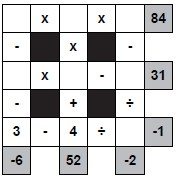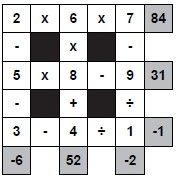Wed, 10 Aug 2022

# Maths Square

Maths Square Instructions:
Here's how to play Maths Square:
Place the numbers from 1 - 9 exactly once into the grid in order to complete the sums.

Sums should be performed from left to right or from top to bottom as relevant. Do NOT perform sums in strict mathematical order (eg multiplication before addition) but simply follow the sum across or down.

Maths squares are a very popular number puzzle. Whilst puzzles that involve maths put some people off, the small size and appealing nature of these puzzles mean that they are very popular and can really help improve mental arithmetic over time.

Puzzles come in a wide range of difficulty levels. Many puzzles come with some givens - that is, numbers placed into the grid at the start. If the puzzle you are solving has givens, then it makes sense to focus on sums containing these numbers first. Remember that if you are given two numbers, there are only seven left to place, as every number must be used precisely once in the grid.

There are many different ways of solving these puzzles and you will learn some of these over time when solving.

Rows or columns where both parts are an addition or both parts are a multiplication are particularly useful, since these can be performed either way around to get to the same solution. For instance 4 + 2 + 3 is the same as 4 + 3 + 2, so you can use this fact quite often to help you make solving progress. Imagine that you know which two numbers must be placed in a sum that is all addition or all multiplication: you can then remove these as candidates for any other sums in the grid, which can be very helpful when solving.

It is also worth thinking about various combinations such as which numbers can add / multiply together to give odd / even answers - spotting whether an answer sum is odd, for instance, can often help you too.

All maths square puzzles have a single solution, and whilst they can be hard to get started on, particularly with the trickier puzzles, you won't have to make blind guesses to solve maths square puzzles.

### Related puzzles

Last updated: 06 Feb 2015

Sample puzzle:Sample solution:View a sample Maths Square PDF

Play Maths Square Puzzles:

If you would like to play maths square puzzles, then you can download and print a PDF magazine of them in our Maths Square Magazine. If you prefer to buy a printed publication, take a look at our Book of Maths Squares.

Publish Maths Square Puzzles: